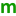# Forward Selection

1. All blocks and forced terms are put into the model.

2. The remaining terms with an “” next to them are considered.

3. Each term is checked, adding them one at a time to the model. The term that improves the criterion the most is added to the model.

4. Terms not yet in the model are checked until no further improvements can be found given the terms already in the model.

Forward works well when the best model is a small subset of the available terms (with an M next to them).

# Backward Elimination

1. All blocks and forced terms are put into the model.

2. The remaining terms with an “” next to them are considered.

3. All remaining terms are added to the model simultaneously.

4. Terms are removed one at a time.

5. The best one-term-smaller model for the selected criterion is kept.

6. If no one-term-smaller model improves the criterion score the elimination process stops, otherwise use the model found in step 5 and return to step 4.

Backward works well when the best model is a large subset of the available terms (with an “” next to them) and there are extra degrees of freedom in the design.

# Step-Wise Regression

Step-wise regression is a combination of forward and backward regressions, starting with two forward steps.

1. All blocks and forced terms are put into the model.

2. The remaining terms with an “” next to them are considered.

3. The term that improves the criterion the most is added to the model.

4. Terms not yet in the model are checked.

5. If a term is found that further improves the criterion it is added. If not the algorithm stops.

When two or more terms are in the model, backward steps are added.

6. Terms are removed one at a time.

7. The best one-term-smaller model that also improves the criterion from step 5 is kept. Return to step 4.

8. If no one-term-smaller model improves the criterion score return to step 4.

Note

The coefficient shown is the coefficient at the time the term was added or deleted from the model. This is likely to be different than the coefficient that is generated with the completed model. For designs with categorical factors, the coefficients are not shown.

# All Hierarchical Model Selection

The all hierarchical models procedure differs from the directional (forward and backward) selection methods. Rather than considering terms one at a time, all hierarchical models are compared simultaneously and the best one is selected. The procedure works as follows:

1. All blocks and forced terms are put into the model.

2. The remaining terms with an “” next to them are considered.

3. The best model of each size (with equal numbers of coefficients) is determined using R-squared and put into a candidate set.

4. The model from the candidate set with the best criterion score is selected.

5. One Backward + p-value step is done to clean up a lingering, insignificant, non-hierarchical term.

All Hierarchical Model Selection can take a long amount of time to complete for designs with 8 or more factors. The search can be manually stopped (Canceled) early and the best model found so far will be presented.

References

• Brusco, Cradit, and Steinly. An exact algorithm for hierarchically well-formulated subsets in second-order polynomial regression. Technometrics, 51(3):306–315, 2009.